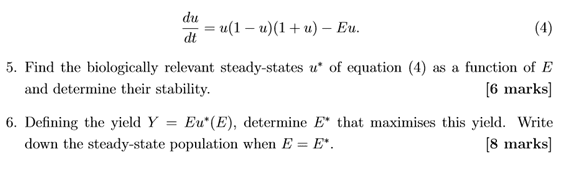• MHB
mt91I've got a question here which I'm really unsure what the wording is asking me to do, I've calculated (5), so worked out the steady states. However question 6 has really thrown me off with it's wording, any help would be appreciated.

HOI
A "steady state" solution to a differential equation is a constant solution. Since the derivatives of a constant are 0, for a "steady state" solution du/dt= 0. For this problem that means u(1- u)(1+ u)- Eu= 0. Factoring out "u", u[(1- u)(1+u)- E]= u[1- u^2- E]= 0. Either u= 0 or u^2= 1- E so the "steady state" solutions are u*(E)= 0 and u*(E)= sqr{1- E}. Is that what you got for (5)?

Problem (6) asks about the "yield" which is defined as Y= Eu*(E) where u* is a "steady state solution". Since the steady state solutions are u*(E)= 0 and u*(E)= sqrt(1- E), either Y= E(0)= 0 or Y= E sqrt(1- E)= sqr(E^2- E^3). The first is identically equal to 0 so cannot be maximized. To find the maximum of the second, set the derivative equal to 0.

Y= sqrt(E^2- E^3)= (E^2- E^3)^(1/2). Y'= (1/2)(E^2- E^3)^(-1/2)(2E- 3E^2)= 0. That is equivalent to 3E^2- 2E= E(3E- 2)= 0 so E= 0 or E= 2/3. Again, E= 0 cannot give a maximum (it gives a minimum) so E*= 2/3.

Joppy
MHB
A "steady state" solution to a differential equation is a constant solution. Since the derivatives of a constant are 0, for a "steady state" solution $\frac{du}{dt}= 0$. For this problem that means $u(1- u)(1+ u)- Eu= 0$. Factoring out "u", $u[(1- u)(1+u)- E]= u[1- u^2- E]= 0$. Either $u= 0$ or $u^2= 1- E$ so the "steady state" solutions are $u^*(E)= 0$ and $u^*(E)= \sqrt{1- E}$. Is that what you got for (5)?

Problem (6) asks about the "yield" which is defined as $Y= Eu^*(E)$ where u* is a "steady state solution". Since the steady state solutions are $u^*(E)= 0$ and $u^*(E)= \sqrt(1- E)$, either $Y= E(0)= 0$ or $Y= E \sqrt(1- E)= \sqrt(E^2- E^3)$. The first is identically equal to 0 so cannot be maximized. To find the maximum of the second, set the derivative equal to 0.

$Y= \sqrt(E^2- E^3)= (E^2- E^3)^{1/2}$. $Y'= (1/2)(E^2- E^3)^(-1/2)(2E- 3E^2)= 0$. That is equivalent to $3E^2- 2E= E(3E- 2)= 0$ so $E= 0$ or $E= 2/3$. Again, $E= 0$ cannot give a maximum (it gives a minimum) so $E^*= 2/3$.